# How to Calculate Average Ignore Non-Numeric Values and Errors

In daily work we often need to calculate the average of some numbers in a range for further analysis. Thus, we need to know some basic functions of calculate average in Excel. From now on, we will introduce you some basic functions like AVERAGE, AVERAGEIF, and show you some common formulas to calculate average value.

In this article, we will let you know how to calculate average by AVERAGE function easily and the way to calculate average based on a given criteria by AVERAGEIF function. We will introduce you the syntax, arguments, and basic usage about the two functions, and let you know the working process of our created formulas.

## EXAMPLE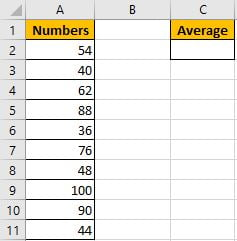Refer to “Numbers” column, some numbers are listed in range A2:A11. C2 is used for entering a formula which can calculate the average of numbers in range A2:A11. In fact, Excel has amount of built-in functions and they can execute most simple calculations properly, and AVERAGE is one of the most common used functions.

In C2, enter “=AVERAGE(A2:A11)”.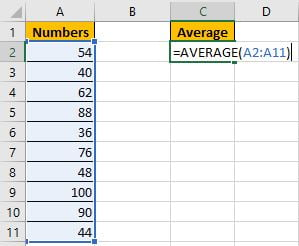Then press Enter, AVERAGE function returns 63.8.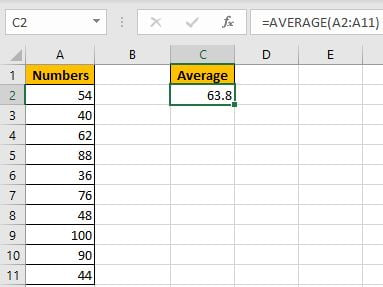You can adjust decimal places by “Increase Decimal” or “Decrease Decimal” in “Number” section.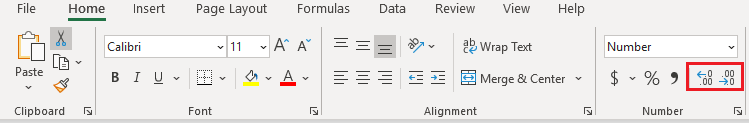But if some errors or blank or non-numeric values exist in the list, how can we only calculate average for numbers ignoring the invalid values?  See example below.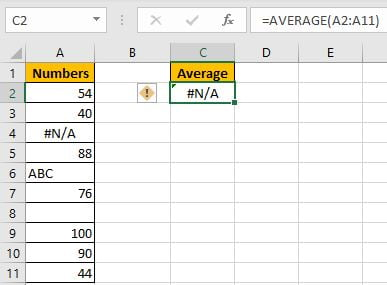We get an error when calculating average for range A2:A11, the difference is there are some invalid values in the list. To calculate average ignoring the errors, that means we need to calculate average of numbers in a range with one or more criteria, thus, we can apply AVERGAEIF function instead of basic AVERAGE function.

## CREATE A FORMULA with AVERAGEIF FUNCTION

### 1. STEPS

Step 1: In C2, enter the formula =AVERAGEIF(A2:A11,”>0″).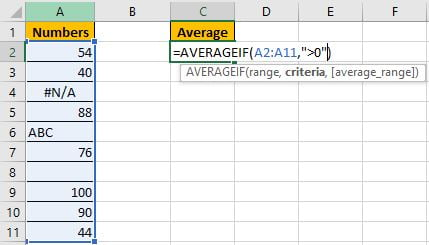You can also name range “Numbers” for A2:A11, then you can enter =AVERAGEIF(Numbers,”>0″).

Step 2: Press Enter after typing the formula.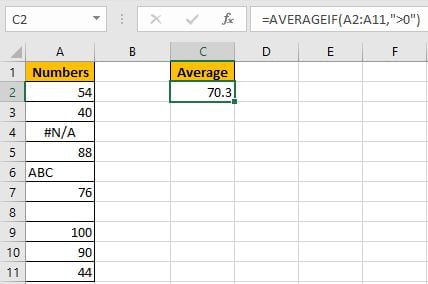Ignore the improper cells, and only calculate the average of numbers 54, 40, 88, 76, 100, 90 and 44, total 7 numbers. (54+40+88+76+100+90+44)/7=70.3. The formula works correctly.

### 2. FUNCTION INTRODUCTION

AVERAGEIF function is AVERAGE+IF. It returns the average of some numbers in a range based on given criteria.

Syntax:

`=AVERAGEIF(range, criteria, [average_range])`

It supports wildcards like asterisk ‘*’ and question mark ‘?’, also supports logical operators like ‘>’,’<’. If wildcards or logical operators are required, they should be enclosed into double quotes (““), in this case we entered “>0” to show the criteria.

### 3. ALL ARGUMENTS

AVERAGEIF – RANGE

This example is very simple, we have only one list. A2:A11 is criteria range and average range.

In the formula bar, select “A2:A11”, press F9, values in this range are expanded in an array.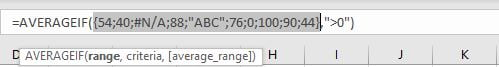If average range=criteria range, average range can be omitted.

AVERAGEIF – CRITERIA

Obviously, the criteria in our case is “>0”, this condition can filter numbers from criteria range.

### 4. HOW THE FORMULA WORKS

After expanding values, the formula is displayed as:

=AVERAGEIF({54;40;#N/A;88;”ABC”;76;0;100;90;44},”>0″)

Note: blank cell is recorded as 0 in the array.

Because of criteria “>0”, so invalid cells are ignored. Replace all invalid values with 0. Keep all numbers.

{54;40;#N/A;88;”ABC”;76;0;100;90;44} -> {54;40;0;88;0;76;0;100;90;44}

Now this new array only contains numbers. We can calculate the average now.

## SUMMARY

1. AVERAGE function is used for returning the average of some numbers in Excel.

2. AVERAGEIF function returns the average of some numbers refer to given criteria.

3. For argument, if range=average range, average range can be omitted.

4. AVERAGEIF can support wildcards, logical operators.

### Related Functions

• Excel AVERAGE function
The Excel AVERAGE function returns the average of the numbers that you provided.The syntax of the AVERAGE function is as below:=AVERAGE (number1,[number2],…)….
• Excel AVERAGEIF function
The Excel AVERAGEAIF function returns the average of all numbers in a range of cells that meet a given criteria.The syntax of the AVERAGEIF function is as below:= AVERAGEIF (range, criteria, [average_range])….

Related Posts

If Cell is This Value or That Value

IF function is frequently used in Excel worksheet to return you expect “true value” or “false value” based on the result of logical test. If you want to see if a cell is A or B, and if one of ...

If Value is Greater Than A Certain Value

IF function is frequently used in Excel worksheet to return you expect “true value” or “false value” based on the logical test result. If you want to see if a value in one cell is greater than a specific value, ...

If Cell is Not Blank

IF function is frequently used in Excel worksheet to return you expect “true value” or “false value” based on the result of created logical test. If you want to see if a cell is blank or not, and leave some ...

If Cell is Blank

IF function is frequently used in Excel worksheet to return you expect “true value” or “false value” based on the result of created logical test. If you want to see if a cell is blank or not, and leave some ...

If Cell Equals Certain Text String

IF function is frequently used in Excel worksheet to return you expect “true value” or “false value” based on the result of created logical test. If you want to see if cell equals a certain text string like “Win”, you ...

If Cell Contains Either Text1 or Text2

IF function is frequently used in Excel worksheet to return “true value” or “false value” based on the logical test result. If you want to see if cell contains certain substring1 like “abc” or substring2 like “def”, and returns true ...

If Cell Contains Certain Text OR Equals Certain Text

IF cell equals certain text IF function is frequently used in Excel worksheet to return “true value” or “false value” based on the logical test result. If you want to test values to see if they equal certain text like ...

VLOOKUP From Another Sheet Not Working

In the previous post, you should know that how to fix or remove the #N/A error when using VLOOKUP formula to lookup value from another sheet. And this post will show you reasons why your VLOOKUP formula is not working ...

If Cell Begins with One of Three Supplied Characters

If you want to test values to see if they begin with some given specific characters like “x”, ”y”, or “z”, you can create a formula with COUNTIF and SUM functions to return results. EXAMPLE You can see “TRUE” or ...

Fix #N/A Error For VLOOKUP From Another Sheet

This post will show you how to fix the #N/A error why it occurs when you extract values from another sheet using VLOOKUP function in Excel 2016,2013,2010 or other Excel versions. How can you correct a #N/A error in VLOOKUP ...

Sidebar